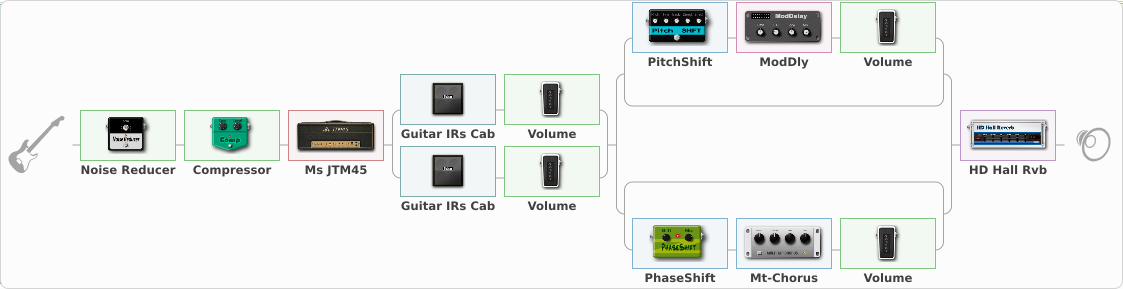# APS MJt Clean

Discussion in 'ToneLib-GFX presets' started by Audio Pirate Studio, Apr 25, 2020.

1. ### Audio Pirate StudioNew Member

APS MJt Clean

Preset name: APS MJt Clean

Atypical clean from a JTM45 with stereo image effects.
Best use with single coil or low gain pick up. It have a break up with humbucker that is possible to control with volume pot.

Effects chain:Effect: "Noise Reducer" (Dynamics / Filter), active - "yes"
{
"Sens" = 60
"Mode" = Soft
}

Effect: "Compressor" (Dynamics / Filter), active - "yes"
{
"Sense" = 33
"Level" = 63
}

Effect: "Ms JTM45" (Amp simulators), active - "yes"
{
"Gain" = 20
"Bass" = 56
"Middle" = 61
"Treble" = 69
"Presence" = 73
"Master" = 73
"Level (dB)" = 8
}

Effect: "Splitter" (Dynamics / Filter), active - "yes"
{
"A-Bypass" = Off
"A-Pan" = 0
"A-Level" = 55
"B-Bypass" = Off
"B-Pan" = 0
"B-Level" = 55

'A' branch:
{

Effect: "Guitar IRs Cab" (Cabinets), active - "yes"
{
"Model" = Fender Twin Rev (2x12")
"Mic Position" = Edge
"Mic Distance" = Near
"Low Cut (Hz)" = 70
"Hi Cut (kHz)" = 20.0
"Mix" = 97
"Level (dB)" = 11
}

Effect: "Volume" (Dynamics / Filter), active - "yes"
{
"Pedal Pos." = 100
"Pan" = -17
}
}
'B' branch:
{

Effect: "Guitar IRs Cab" (Cabinets), active - "yes"
{
"Model" = Vox AC30 (2x12")
"Mic Position" = Edge
"Mic Distance" = Near
"Low Cut (Hz)" = 71
"Hi Cut (kHz)" = 20.0
"Mix" = 99
"Level (dB)" = 11
}

Effect: "Volume" (Dynamics / Filter), active - "yes"
{
"Pedal Pos." = 100
"Pan" = 18
}
}
}

Effect: "Splitter" (Dynamics / Filter), active - "yes"
{
"A-Bypass" = Off
"A-Pan" = 0
"A-Level" = 55
"B-Bypass" = Off
"B-Pan" = 0
"B-Level" = 55

'A' branch:
{

Effect: "Splitter" (Dynamics / Filter), active - "yes"
{
"A-Bypass" = Off
"A-Pan" = 0
"A-Level" = 55
"B-Bypass" = Off
"B-Pan" = 0
"B-Level" = 55

'A' branch:
{

Effect: "PitchShift" (Modulation / Sfx), active - "yes"
{
"Pitch" = 0
"Fine" = -10
"Tracking" = 111
"Direct" = 0
"Effect" = 85
}

Effect: "ModDly" (Delay), active - "yes"
{
"Time" = Note sync, 1/8
"Feedback" = 46
"Tone" = 25
"Speed" = 4.2
"Mix" = 100
}

Effect: "Volume" (Dynamics / Filter), active - "yes"
{
"Pedal Pos." = 63
"Pan" = -23
}
}
'B' branch:
{
}
}
}
'B' branch:
{

Effect: "Splitter" (Dynamics / Filter), active - "yes"
{
"A-Bypass" = Off
"A-Pan" = 0
"A-Level" = 55
"B-Bypass" = Off
"B-Pan" = 0
"B-Level" = 55

'A' branch:
{
}
'B' branch:
{

Effect: "PhaseShift" (Modulation / Sfx), active - "yes"
{
"Shift" = 34
"Mix" = 81
}

Effect: "Mt-Chorus" (Modulation / Sfx), active - "yes"
{
"Speed" = 4.5
"Depth" = 65
"Time" = 3.5
"Mix" = 100
}

Effect: "Volume" (Dynamics / Filter), active - "yes"
{
"Pedal Pos." = 55
"Pan" = 9
}
}
}
}
}

Effect: "HD Hall Rvb" (Reverb), active - "yes"
{
"Time" = 6.9
"PreLPF" = 42
"PreDelay" = 107
"HiDamp" = 53
"LoGain" = 1.2
"Mix" = 21
}

Note: You will need to download and install the ToneLib-GFX software to use the preset.

#### Attached Files:

• ###### APS_MJt_Clean.tlgfx
File size:
1.5 KB
Views:
3,009
SEMENOV and sonar72 like this.